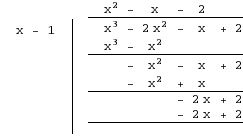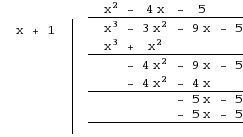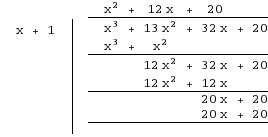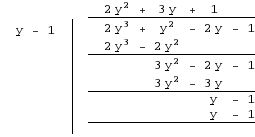Related Articles
Class 9 NCERT Solutions – Chapter 2 Polynomials – Exercise 2.4
• Difficulty Level : Expert
• Last Updated : 01 Apr, 2021

### Question 1. Determine which of the following polynomials has (x + 1) as a factor:

(i) x3+x2+x+1

Solution:

p(x) = x3 + x2 + x + 1

Let x+1 be the factor of p(x)
Then x = -1 will be the zero of p(x)
value of p(-1) should be 0
Checking,
=> p(-1) = (-1)3 + (-1)2 + (-1) + 1
=> -1 + 1 -1 + 1
=> 0
As p(-1)=0 so (x + 1) is a factor of p(x).

(ii) x4+x3+x2+x+1

Solution:

p(x) = x4+x3+x2+x+1
Let x+1 be the factor of p(x)
Then x = -1 will be the zero of p(x)
value of p(-1) should be 0
Checking,
=> p(-1) = (-1)4 + (-1)3 + (-1)2 + (-1) + 1
=> – 1 + 1 – 1 + 1 -1
=> -1
=> -1 ≠ 0
As p(-1) ≠ 0 so (x + 1) is not a factor of p(x).

(iii) x4+3x3+3x2+x+1

Solution:

p(x) = x4+3x3+3x2+x+1
Let x+1 be the factor of p(x)
Then x = -1 will be the zero of p(x)
value of p(-1) should be 0
Checking,
=> p(-1) = (-1)4 + 3(-1)3 + 3(-1)2 + (-1) + 1
=> 1 – 3 + 3 – 1 + 1
=> -1
=> -1 ≠ 0
As p(-1) ≠ 0 so (x + 1) is not a factor of p(x).

(iv) x3 – x2– (2+√2)x +√2

Solution:

p(x) = x3 – x2– (2+√2)x +√2
Let x+1 be the factor of p(x)
Then x = -1 will be the zero of p(x)
value of p(-1) should be 0
Checking,
=> p(-1) = (-1)3 – (-1)2– (2+√2)(-1) +√2
=> -1 – 1 + 2 + √2 + √2
=> 2√2
=> 2√2 ≠ 0
As p(-1) ≠ 0 so (x + 1) is not a factor of p(x).

### Question 2. Use the Factor Theorem to determine whether g(x) is a factor of p(x) in each of the following cases:

(i) p(x) = 2x3+x2–2x–1, g(x) = x+1

Solution:

p(x) = 2x3+x2– 2x–1
g(x) = x + 1
By Factor Theorem we know that if x + 1 is a factor of p(x)
Then value of p(-1) should be 0
Checking,
=> p(-1) = 2(-1)3 + (-1)2 – 2(-1) -1
=> -2 + 1 + 2 – 1
=> 0
As p(-1) = 0 therefore (x + 1) is a factor of 2x3 + x2 – 2x – 1

(ii) p(x) = x3+3x2+3x+1, g(x) = x+2

Solution:

p(x) = x3+3x2+3x+1
g(x) = x + 2
By Factor Theorem we know that if x + 2 is a factor of p(x)
Then value of p(-2) should be 0
Checking,
=> p(-2) = (-2)3 + 3(-2)2 + 3(-2) +1
=> -8 + 12 – 6 + 1
=> -1
=> -1 ≠ 0
As p(-2) ≠ 0 therefore (x + 2) is not a factor of x3 + 3x2 +3x + 1

(iii) p(x)=x3– 4x2+x+6, g(x) = x – 3

Solution:

p(x) = x3– 4x2+x+6
g(x) = x – 3
By Factor Theorem we know that if x – 3 is a factor of p(x)
Then value of p(3) should be 0
Checking,
=> p(3) = (3)3 – 4(3)2 + 3 + 6
=> 27 – 36 + 3 + 6
=> 0
As p(3)=0 so (x – 3) is a factor of p(x).

### Question 3. Find the value of k, if x–1 is a factor of p(x) in each of the following cases:

(i) p(x) = x2+x+k

Solution:

p(x) = x2 + x + k
By Factor Theorem,
As x-1 is a factor of p(x)
then x = 1 is the zero of p(x)
Therefore p(1) = 0
=> p(1) = (1)2 + 1 + k
=> 1 + 1 + k = 0
=> 2 + k = 0
=> k = -2

(ii) p(x) = 2x2+kx+√2

Solution:

p(x) = 2x2 + kx + √2
By Factor Theorem,
As x-1 is a factor of p(x)
then x = 1 is the zero of p(x)
Therefore p(1) = 0
=> p(1) = 2(1)2 + k(1) + √2
=> 2 + k + √2 = 0
=> 2 + √2 + k = 0
=> k = – (2 + √2)

(iii) p(x) = kx2–√2x+1

Solution:

p(x) = kx2 – √2x + 1
By Factor Theorem,
As x-1 is a factor of p(x)
then x = 1 is the zero of p(x)
Therefore p(1) = 0
=> p(1) = k(1)2 – √2(1) + 1
=> k – √2 + 1 = 0
=> k = √2 – 1

(iv) p(x) = kx2–3x+k

Solution:

p(x) = kx2 -3x + k
By Factor Theorem,
As x-1 is a factor of p(x)
Then x = 1 is the zero of p(x)
Therefore, p(1) = 0
=> p(1) = k(1)2 – 3(1) + k
=> k – 3 + k = 0
=> 2k – 3 = 0
=> k = 3/2

### Question 4. Factorize:

(i) 12x2–7x+1

Solution:

p(x) = 12x2 – 7x + 1
Using splitting the middle term method,
We need to find a pair of numbers whose sum is -7x
and product is 12x2
-7x can be written as the sum of -3x and -4x
12x2 can be written as the product of -3x and -4x
=> 12x2 – 7x + 1
=> 12x2 -3x -4x +1
=> 3x(4x -1) -1(4x -1)
=> (3x – 1)(4x – 1) are the factors of 12x2 – 7x + 1

(ii) 2x2+7x+3

Solution:

p(x) = 2x2 + 7x + 3
Using splitting the middle term method,
We need to find a pair of numbers whose sum is 7x
and product is 6x2
7x can be written as the sum of 1x and 6x
6x2 can be written as the product of 1x and 6x
=> 2x2 + 7x + 3
=> 2x2 + 1x + 6x + 3
=> 2x(x + 3) + 1(x + 3)
=> (2x + 1)(x + 3) are the factors of 2x2 + 7x + 3

(iii) 6x2+5x-6

Solution:

p(x) = 6x2 + 5x – 6
Using splitting the middle term method,
We need to find a pair of numbers whose sum is 5x
and product is -36x2
5x can be written as the sum of 9x and -4x
-36x2 can be written as the product of 9x and -4x
=> 6x2 + 5x – 6
=> 6x2 + 9x – 4x – 6
=> 3x(2x + 3) – 2(2x + 3)
=> (3x – 2)(2x + 3) are the factors of 6x2 + 5x – 6

(iv) 3x2–x–4

Solution:

p(x) = 3x2 – x – 4
Using splitting the middle term method,
We need to find a pair of numbers whose sum is -x
and product is -12x2
-x can be written as the sum of -4x and 3x
-12x2 can be written as the product of -4x and 3x
=> 3x2 – x – 4
=> 3x2 – 4x + 3x – 4
=> 3x(x + 1) – 4(x + 1)
=> (3x – 4)(x + 1) are the factors of 3x2 – x – 4

### Question 5. Factorize:

(i) x3–2x2–x+2

Solution:

p(x) = x3– 2x2– x + 2
Factors of 2 are ±1 and ± 2
Using Hit and Trial Method
p(1) = (1)3 – 2(1)2 – (1) + 2
p(1) = 1 – 2 – 1 + 2
p(1) = 0
Therefore, (x – 1) is a factor of x3 – 2x2 – x + 2
Performing Long Division :Quotient : x2 – x – 2 , Remainder : 0

Dividend = Divisor × Quotient + Remainder
=>p(x) = (x – 1)(x2 – x – 2)
=> solving (x2 – x -2)
=> using Splitting the middle term method
=> x2 – 2x + x – 2
=> x(x – 2) + 1(x – 2)
=> (x + 1)(x – 2)
=>(x – 1)(x + 1)(x – 2) are the factors of p(x)

(ii) x3–3x2–9x–5

Solution:

p(x) = x3–3x2–9x–5
Factors of -5 are ±1 and ± 5
Using Hit and Trial Method
let x = 1
p(1) = (1)3 – 3(1)2 – 9(1) – 5
p(1) = 1 – 3 – 9 -5
p(1) = -16
p(1) ≠ 0
let x = -1
p(-1) = (-1)3 – 3(-1)2 – 9(-1) – 5
p(-1) = -1 – 3 + 9 – 5
p(-1) = -9 + 9
p(-1) = 0
Therefore, (x + 1) is a factor of p(x)
Performing Long Division :Quotient : x2 – 4x – 5, Remainder : 0

Dividend = Divisor × Quotient + Remainder
=>p(x) = (x + 1)(x2 – 4x – 5)
=> solving (x2 – 4x – 5)
=> using splitting the middle term method
=> x2 -5x + x – 5
=> x(x – 5) + 1(x – 5)
=> (x + 1)(x – 5)
=>(x + 1)(x + 1)(x – 5) are the factors of p(x)

(iii) x3+13x2+32x+20

Solution:

p(x) = x3+13x2+32x+20
=> Factors of 20 are ±1, ±2, ±4, ±5, ±10 and ±20
Using Hit and Trial Method
let x = 1
p(1) = (1)3 + 13(1)2 + 32(1) + 20
p(1) = 1 + 13 + 32 + 20
p(1) = 66
p(1) ≠ 0
let x = -1
p(-1) = (-1)3 + 13(-1)2 + 32(-1) + 20
p(-1) = -1 + 13 – 32 + 20
p(-1) = -33 + 33
p(-1) = 0
Therefore, (x + 1) is a factor of p(x)
Performing Long Division :Quotient : x2 + 12x + 20, Remainder : 0

Dividend = Divisor × Quotient + Remainder
=> p(x) = (x + 1)(x2 + 12x + 20)
=> solving x2 + 12x + 20
=> using Splitting the middle term method
=> x2 + 10x + 2x + 20
=> x(x + 10) + 2(x + 10)
=> (x + 2)(x + 10) are the factors of x2 + 12x + 20
=> (x + 1)(x + 2)(x + 10) are the factors of p(x)

(iv) 2y3+y2–2y–1

Solution:

p(y) = 2y3+y2–2y–1
=> Factors of -1 are ±1
Using Hit and Trial Method
let x = 1
p(1) = 2(1)3 + (1)2 – 2(1) – 1
p(1) = 2 + 1 -2 – 1
p(1) = 0
Therefore, (y – 1) is a factor of p(y)
Performing Long Division :Quotient : 2y2 + 3y + 1, Remainder : 0

Dividend = Divisor × Quotient + Remainder
=> p(y) = (y – 1)(2y2 + 3y + 1)
=> solving 2y2 + 3y +1
=> using splitting the middle term method
=> 2y2 + 2y +y +1
=> 2y(y + 1)+1(2y + 1)
=>(2y + 1)(2y + 1) are the factors of 2y2 + 3y + 1
=> (y – 1)(2y + 1)(2y + 1) are the factors of p(y)

My Personal Notes arrow_drop_up